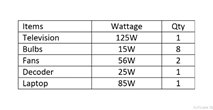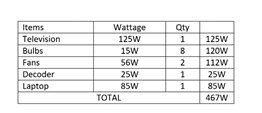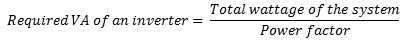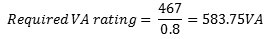# How to calculate the size of inverter needed for your home

## WHAT SIZE OF INVERTER DO I NEED FOR MY HOME?

A lot of persons want a power backup or solar system but don’t know how to calculate the energy requirement of their homes. As a side note, you should know that Inverters supply peak /surge power and typical (continuous rating) power. What do these mean?

Appliances such as those with electric motors need high startup surge. This can be referred to as surge power which is the maximum power an inverter can supply for a short time.

When a refrigerator is turned on, the power it ‘pulls’ at first is referred to as SURGE POWER. The power that is supplied on a steady basis afterwards is called CONTINUOUS RATING or typical power.

Moving ahead, let’s calculate the inverter size you need for your home in the following steps:

### 1.  CALCULATE THE TOTAL POWER NEEDEDFirst, what load do you intend to power? Let’s say 1TV (125W), 8 bulbs (15W each), 2 Fans (56W each), 1 Decoder (25W) and 1 Laptop (85W).The total power needed will be calculated as “Power = Wattage of appliance x Quantity.” Therefore, you’ll need a total power of (125×1) + (15×8) bulbs + (56×2) fans + (25×1) decoder + (85×1) = 467W.

### 2. CALCULATE THE INVERTER’S REQUIRED CAPACITY

Now, let’s calculate the inverter’s required capacity, i.e the Volt-Ampere rating. In an ideal condition, an inverter would operate with 100% efficiency. Most inverters have an efficiency of between 60% and 80%. This efficiency can also be referred to as the power factor of an inverter. For our calculations, we would use a power factor of 0.8. Hence,Power supplied (or VA rating of the inverter) = Power consumed by equipment in watts / Power factor

Recall, the total power consumed by your home (total wattage) – 467W.
Power factor = 0.8

Therefore, required VA rating of inverter = (467/0.8) = 583.75VA.This is approximately a 0.6kVA (600VA).

Using a 0.6kVA inverter will be inappropriate considering surge power and the probability of adding a few small appliances to the system. A 0.8kVA inverter will be suitable for your home. 😀

Are you having any difficulty in calculating the size of inverter needed for your home? Do you have any questions? Please comment or Contact us!

Do you need an inverter/solar system? We are just the solution you need.

••